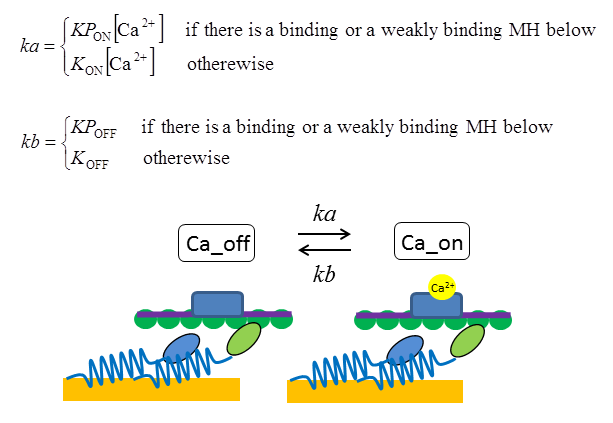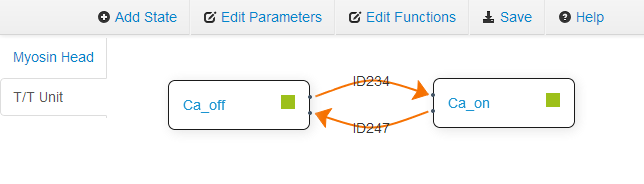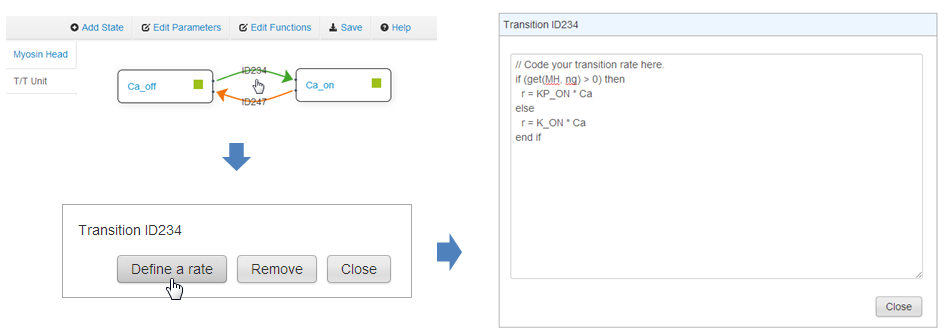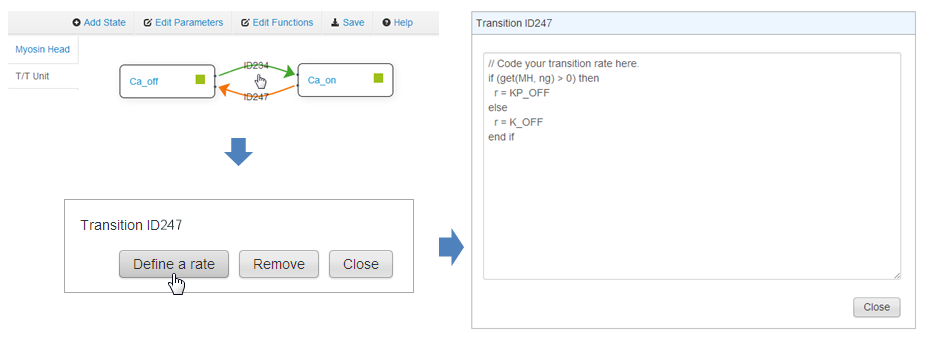## Defining transitions of the T/T units

Here we assume that the calcium ion affinity to the troponin in the T/T unit increases if there is at least one myosin head binding (XB_PreR, XB_PostR) or weakly binding (P_XB) to the actin filament within the segment of the T/T unit. We also assume that the transition rate from Ca_off to Ca_on is proportional to the calcium ion concentration. The dependence of the transitions to the myosin head states below the T/T unit can be implemented by calling the function ng defined in Defining new parameters and functions of the force regulatory model### Constructing the transition paths

Go to the T/T Unit edit page by clicking on T/T Unit tab if you are not in the T/T Unit edit page. Construct the transition paths from Ca_off to Ca_on and from Ca_on to Ca_off. For example, the path from Ca_off to Ca_on can be made by clicking on the green square button in the state box Ca_off and dragging the orange arrow to the state box Ca_on and releasing the mouse button.### Defining transition rate from Ca_off to Ca_on

Click on the arrow from Ca_off to Ca_on. Click on the Define a rate button. Define the rate "r" in the Transition dialog. Here "r" is the transition rate that means how many times this transition occurs in a second on average.Input the following codes in the Transition dialog. We can use an if-statement. Here KP_ON and K_ON are the parameters given in Your Custom Parameter dialog. "get(MH, ng)" returns the maximal　value of ng for the myosin heads which are located below the T/T unit. If there is no myosin head below the segment, the zero value is returned by the get command. "MH" in the first argument indicates to refer a function defined for the myosin head states. "Ca" is the calcium ion concentration given by the XB Modeler.

```if (get(MH, ng) > 0) then
r = KP_ON * Ca
else
r = K_ON * Ca
end if
```

After writing the code, click on the Close button.

### Defining transition rate from Ca_on to Ca_off

Click on the arrow from Ca_on to Ca_off. Click on the Define a rate button. Define the rate "r" in the Transition dialog.Input the following codes in the Transition dialog. We can use an if-statement. Here KP_OFF and K_OFF are the parameters given in the Your Custom Parameter form. "get(MH, ng)" returns the maximal　value of ng for the myosin heads which are located below the T/T unit.

```if (get(MH, ng) > 0) then
r = KP_OFF
else
r = K_OFF
end if
```